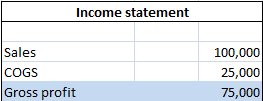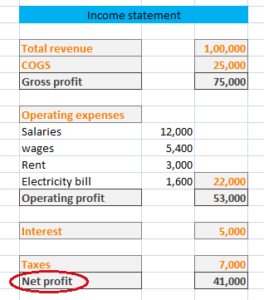## Meaning of Amalgamation:

Amalgamation is the process in which a new company is formed by dissolving two or more companies. Amalgamation happens in companies running the same line of business or have some synergy in their operations.

For example: Companies ABC Pvt Ltd and XYZ Ltd decide to merge their business and start operating as a new company i.e. PQR Pvt Ltd.

## Effects of amalgamation:

Amalgamation has certain effects on the participating companies. These effects are as follows:

1. ### Existence of existing companies:

The existence of existing companies disappears from market as both the companies are dissolved while formulating a new company.

1. ### Assets and Liabilities of companies:

The new company takes over the assets and liabilities of participating companies.

In amalgamation, the newly formed company get advantages over the merged ones. These advantages include:

1. ### Stronger company:

The assets of companies are collectively used by the newly established company. Thus, it comes into existence as a stronger company from all the merged companies.

1. ### Larger customer base:

As a combined unit, all the amalgamated companies share their customer base which is accumulation of total customer base of merged companies.

1. ### Eliminate competition:

Earlier the companies were competing with each other but after shaping into one they get edge over their competitors.

1. ### Improve managerial effectiveness:

After amalgamation, management of participating companies work together for the growth of newly launched company. It doesn’t only increase their chances of success in market but also makes the decision making process more effective.

The disadvantages of amalgamation of companies are increased liabilities and chances of monopoly.

1. ### Increased liabilities:

On one side, participating companies combined their assets, on other side they carry their accumulated liabilities.

1. ### Chances of monopoly:

When two or more companies share their resources, there are chances that they diminish their competition. This could result in monopoly of company.

## Gross Profit – Meaning and Formula

Gross profit also known as gross income is the excess of net sales over cost of goods sold. Put it simple, gross income is the difference between net revenue (net sale) and cost of goods sold. Gross income accounts for operating expenses, interests and taxes.

### Formula for the calculation of gross profit:

Gross income = Net sales – Cost of goods sold

Net revenue or sales is calculated by subtracting the amount of sales returns, allowances and discount from gross sales.

Net revenue = Gross sales – (Sales returns + Allowances + Discounts)

Cost of goods sold (COGS) refers to direct costs incurred in manufacturing products or providing services and is calculated as

COGS = Opening stock + Purchases – Closing stock

### Example:

From the figures given in income statement of XYZ ltd, gross income can calculated as:

###Gross sales = 100,000

Cost of goods sold = 25,000

Gross income = 1,00,000 – 25,000 = 75,000.

Gross income figure is used in calculation of one of the important profitability ratios known as gross profit margin.

## Net profit – Meaning & Formula

Net profit is the excess of a company’s net revenue (net sales) over its total expenses. For this purpose, total expenses include both direct and indirect expenses as well as interest and taxes.

If a company is liable to pay dividend to preference shareholders then the amount of dividend is also subtracted to get this figure. Here, it should be noted that the dividend paid to equity shareholders is not deducted from net sales.

Net profit is the also known as Profit after tax (PAT).

### Formula for the calculation of net profit:

Net sales – COGS* – Operating expenses – Interest and taxes – Dividend to preference shareholders (If any)

Or

Gross profit – Operating expenses – Interest and taxes – Dividend to preference shareholders (If any)

Or

Operating profit – Interest and Taxes – Dividend to preference shareholders (If any)

*Cost of goods sold (COGS) = Opening stock + Purchases – Closing stock

### Example:

From the figures provided in income statement of XYZ ltd, PAT can be calculated as:Net profit = Gross profit – Operating expenses – Interest and taxes

= 75,000 – 22,000 – 12,000* = 41,000

Or

Operating profit – (Interest and Taxes)

= 53,000 – 12,000* = 41,000

* The given figure is the sum total of Interest and Taxes.

## Net profit margin – Meaning & Formula

Net profit margin is calculated by dividing the amount of net profit by net sale. This is one of the important profitability ratios used to gauge the overall profitability of a firm. This ratio is often used to measure the efficiency of a management to derive profit out of its sale.

Net profit is the amount which is left after deducting all direct and indirect expenses from the amount of total sales.

Net profit = Operating profit – Interest and taxes

In case a company pays dividend to its shareholders then dividend paid to preference shareholders (not to equity shareholders) is also deducted to get the net profit.

### Formula for the calculation of net profit margin:

Net profit ratio = Net profit/ Net revenue

The higher degree of this ratio shows that management is effectively generating profit while incurring lower costs.

## Operating profit margin – Meaning & Formula

Operating profit margin is one of the profitability ratios used to gauge the operating profitability of a firm. This ratio represents the relationship between operating profit and net sale of a firm.

Operating profit is calculated by subtracting the amount of cost of goods sold (COGS) and operating expenses from total sales. This is the profit which includes interest and taxes amount. That’s why, it is also known as ‘Earning before interest and taxes (EBIT)’.

Operating profit margin is also known as ‘Operating margin’.

### Formula for calculation of operating profit margin:

Operating margin is calculated by dividing the amount of operating profit by net revenue (net sale).

Operating profit ratio = Operating profit/ Net sale

As operating profit is calculated by deducting the amount of operating expenses from the amount of gross profit, below formula can also be used in calculation of operating margin

Operating profit ratio = (Gross profit – Operating expenses)/ Net sales

The higher degree of this ratio shows that management is effectively generating profit while incurring lower operating costs.